## Wednesday, June 21, 2017

### Generating a set of random resolutions of all the nodes in a tree with multifurcations

Today, an R-sig-phylo list member asked:

“I am using the ape package to randomly resolve polytomies using 'multi2di' and wondering if there is a way to use this function to get a single output tree file that contains multiple different randomly resolved trees using some number of resamplings?”

This can be done using the phytools function `resolveNode`, which returns all possible resolutions of a given node. We just iterate over all the nodes of the tree pick a random resolution each time.

Here's a function to do that:

``````resolveRandom<-function(tree){
while(!is.binary(tree)){
nodes<-1:tree\$Nnode+Ntip(tree)
Nchildren<-function(node,tree) length(Children(tree,node))
nchilds<-sapply(nodes,Nchildren,tree=tree)
node<-nodes[which(nchilds>2)]
tree<-sample(resolveNode(tree,node),1)[]
}
tree
}
``````

Here's a quick demo:

``````library(phytools)
library(phangorn)
``````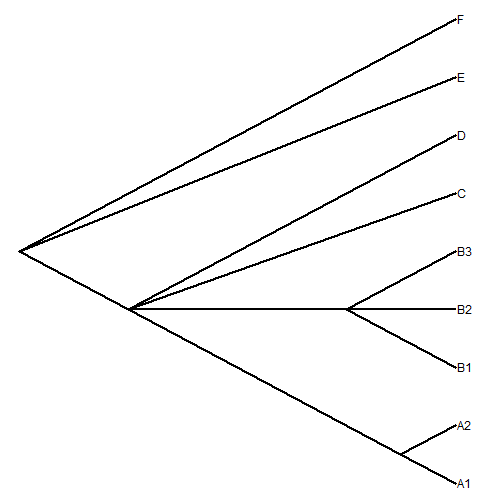``````## now some random resolutions
``````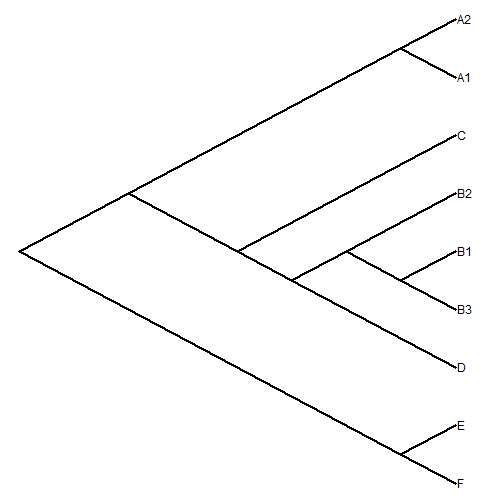``````plotTree(resolveRandom(tree),type="cladogram",nodes="centered")
``````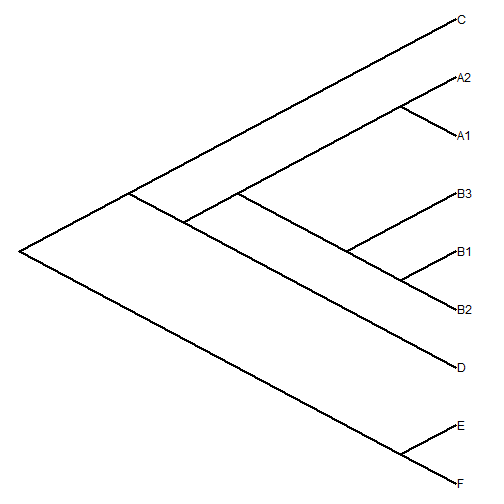``````plotTree(resolveRandom(tree),type="cladogram",nodes="centered")
``````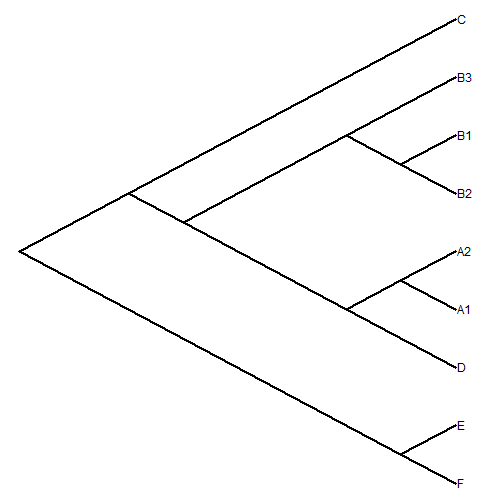``````plotTree(resolveRandom(tree),type="cladogram",nodes="centered")
``````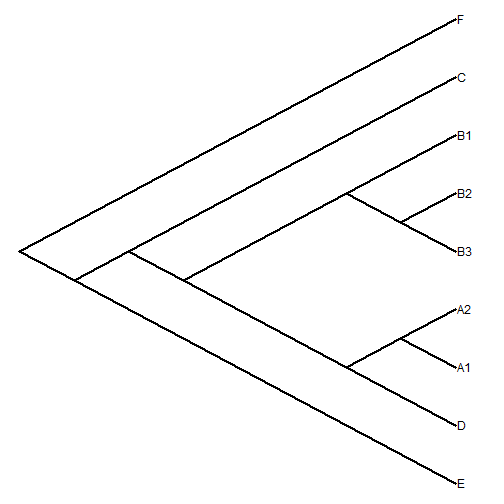We can also generate a set of resolutions using `replicate`:

``````trees<-replicate(36,resolveRandom(tree),simplify=FALSE)
class(trees)<-"multiPhylo"
par(mfrow=c(6,6))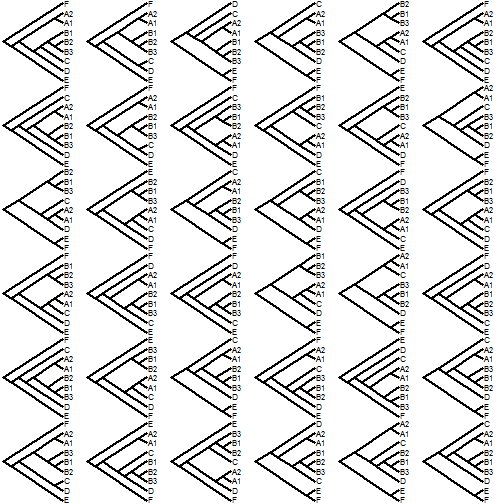1.